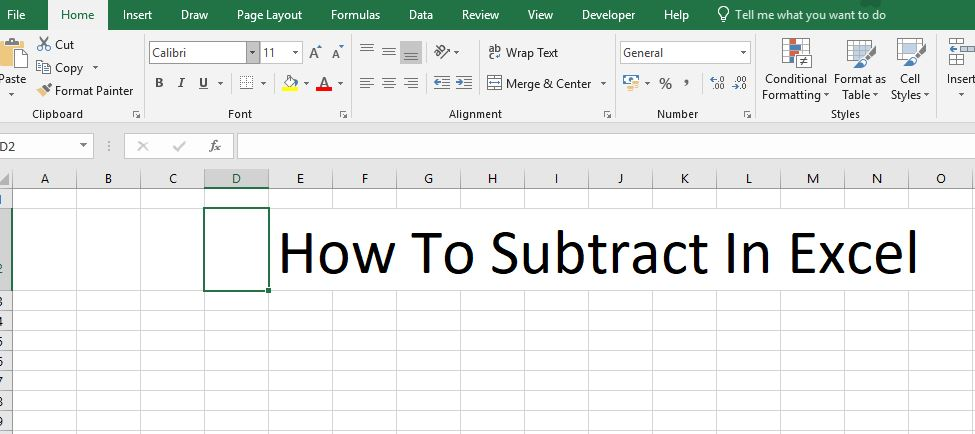# Tag Archives: how to subtract in excel# How to Subtract in Excel

Learn how to subtract in Excel with this valuable how-to guide. This article will walk you through each step of the process from start to finish.

Excel is a powerful program that makes organizing numbers and data easy for anyone. But, learning how to perform even simple functions can be a bit tricky when first starting out. Excel can perform many different functions and one of the most basic is subtraction. Below you will find a complete guide on how to subtract in Excel.

We don’t know why Microsoft didn’t make it but there is no subtract function in Excel. Read the rest